This is “Chemical Compounds”, section 2.1 from the book Principles of General Chemistry (v. 1.0M). For details on it (including licensing), click here.

For more information on the source of this book, or why it is available for free, please see the project's home page. You can browse or download additional books there. To download a .zip file containing this book to use offline, simply click here.

Has this book helped you? Consider passing it on:
Creative Commons supports free culture from music to education. Their licenses helped make this book available to you.
DonorsChoose.org helps people like you help teachers fund their classroom projects, from art supplies to books to calculators.

## 2.1 Chemical Compounds

### Learning Objective

1. To understand the differences between covalent and ionic bonding.

The atoms in all substances that contain more than one atom are held together by electrostatic interactionsAn interaction between electrically charged particles such as protons and electrons.—interactions between electrically charged particles such as protons and electrons. Electrostatic attractionAn electrostatic interaction between oppositely charged species (positive and negative) that results in a force that causes them to move toward each other. between oppositely charged species (positive and negative) results in a force that causes them to move toward each other, like the attraction between opposite poles of two magnets. In contrast, electrostatic repulsionAn electrostatic interaction between two species that have the same charge (both positive or both negative) that results in a force that causes them to repel each other. between two species with the same charge (either both positive or both negative) results in a force that causes them to repel each other, as do the same poles of two magnets. Atoms form chemical compounds when the attractive electrostatic interactions between them are stronger than the repulsive interactions. Collectively, we refer to the attractive interactions between atoms as chemical bondsAn attractive interaction between atoms that holds them together in compounds..

Chemical bonds are generally divided into two fundamentally different kinds: ionic and covalent. In reality, however, the bonds in most substances are neither purely ionic nor purely covalent, but they are closer to one of these extremes. Although purely ionic and purely covalent bonds represent extreme cases that are seldom encountered in anything but very simple substances, a brief discussion of these two extremes helps us understand why substances that have different kinds of chemical bonds have very different properties. Ionic compoundsA compound consisting of positively charged ions (cations) and negatively charged ions (anions) held together by strong electrostatic forces. consist of positively and negatively charged ions held together by strong electrostatic forces, whereas covalent compoundsA compound that consists of discrete molecules. generally consist of moleculesA group of atoms in which one or more pairs of electrons are shared between bonded atoms., which are groups of atoms in which one or more pairs of electrons are shared between bonded atoms. In a covalent bondThe electrostatic attraction between the positively charged nuclei of the bonded atoms and the negatively charged electrons they share., the atoms are held together by the electrostatic attraction between the positively charged nuclei of the bonded atoms and the negatively charged electrons they share. We begin our discussion of structures and formulas by describing covalent compounds. The energetic factors involved in bond formation are described in more quantitative detail in Chapter 8 "Ionic versus Covalent Bonding".

### Note the Pattern

Ionic compounds consist of ions of opposite charges held together by strong electrostatic forces, whereas pairs of electrons are shared between bonded atoms in covalent compounds.

## Covalent Molecules and Compounds

Just as an atom is the simplest unit that has the fundamental chemical properties of an element, a molecule is the simplest unit that has the fundamental chemical properties of a covalent compound. Some pure elements exist as covalent molecules. Hydrogen, nitrogen, oxygen, and the halogens occur naturally as the diatomic (“two atoms”) molecules H2, N2, O2, F2, Cl2, Br2, and I2 (part (a) in Figure 2.1 "Elements That Exist as Covalent Molecules"). Similarly, a few pure elements are polyatomicMolecules that contain more than two atoms. (“many atoms”) molecules, such as elemental phosphorus and sulfur, which occur as P4 and S8 (part (b) in Figure 2.1 "Elements That Exist as Covalent Molecules").

Each covalent compound is represented by a molecular formulaA representation of a covalent compound that consists of the atomic symbol for each component element (in a prescribed order) accompanied by a subscript indicating the number of atoms of that element in the molecule. The subscript is written only if the number is greater than 1., which gives the atomic symbol for each component element, in a prescribed order, accompanied by a subscript indicating the number of atoms of that element in the molecule. The subscript is written only if the number of atoms is greater than 1. For example, water, with two hydrogen atoms and one oxygen atom per molecule, is written as H2O. Similarly, carbon dioxide, which contains one carbon atom and two oxygen atoms in each molecule, is written as CO2.

Figure 2.1 Elements That Exist as Covalent Molecules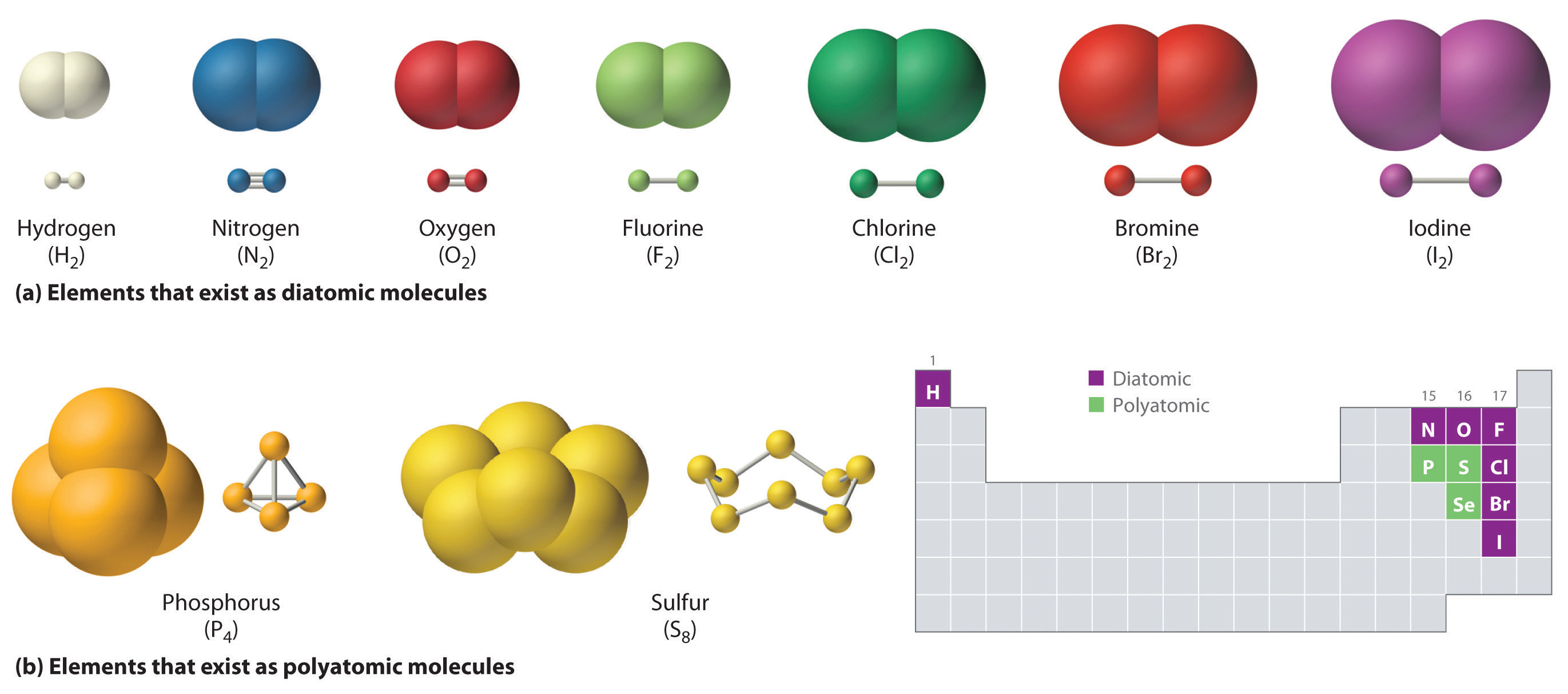(a) Several elements naturally exist as diatomic molecules, in which two atoms (E) are joined by one or more covalent bonds to form a molecule with the general formula E2. (b) A few elements naturally exist as polyatomic molecules, which contain more than two atoms. For example, phosphorus exists as P4 tetrahedra—regular polyhedra with four triangular sides—with a phosphorus atom at each vertex. Elemental sulfur consists of a puckered ring of eight sulfur atoms connected by single bonds. Selenium is not shown due to the complexity of its structure.

Covalent compounds that contain predominantly carbon and hydrogen are called organic compoundsA covalent compound that contains predominantly carbon and hydrogen.. The convention for representing the formulas of organic compounds is to write carbon first, followed by hydrogen and then any other elements in alphabetical order (e.g., CH4O is methyl alcohol, a fuel). Compounds that consist primarily of elements other than carbon and hydrogen are called inorganic compoundsAn ionic or covalent compound that consists primarily of elements other than carbon and hydrogen.; they include both covalent and ionic compounds. In inorganic compounds, the component elements are listed beginning with the one farthest to the left in the periodic table (see Chapter 32 "Appendix H: Periodic Table of Elements"), such as we see in CO2 or SF6. Those in the same group are listed beginning with the lower element and working up, as in ClF. By convention, however, when an inorganic compound contains both hydrogen and an element from groups 13–15, the hydrogen is usually listed last in the formula. Examples are ammonia (NH3) and silane (SiH4). Compounds such as water, whose compositions were established long before this convention was adopted, are always written with hydrogen first: Water is always written as H2O, not OH2. The conventions for inorganic acids, such as hydrochloric acid (HCl) and sulfuric acid (H2SO4), are described in Section 2.5 "Acids and Bases".

### Note the Pattern

For organic compounds: write C first, then H, and then the other elements in alphabetical order. For molecular inorganic compounds: start with the element at far left in the periodic table; list elements in same group beginning with the lower element and working up.

### Example 1

Write the molecular formula of each compound.

1. The phosphorus-sulfur compound that is responsible for the ignition of so-called strike anywhere matches has 4 phosphorus atoms and 3 sulfur atoms per molecule.
2. Ethyl alcohol, the alcohol of alcoholic beverages, has 1 oxygen atom, 2 carbon atoms, and 6 hydrogen atoms per molecule.
3. Freon-11, once widely used in automobile air conditioners and implicated in damage to the ozone layer, has 1 carbon atom, 3 chlorine atoms, and 1 fluorine atom per molecule.

Given: identity of elements present and number of atoms of each

Asked for: molecular formula

Strategy:

A Identify the symbol for each element in the molecule. Then identify the substance as either an organic compound or an inorganic compound.

B If the substance is an organic compound, arrange the elements in order beginning with carbon and hydrogen and then list the other elements alphabetically. If it is an inorganic compound, list the elements beginning with the one farthest left in the periodic table. List elements in the same group starting with the lower element and working up.

C From the information given, add a subscript for each kind of atom to write the molecular formula.

Solution:

1. A The molecule has 4 phosphorus atoms and 3 sulfur atoms. Because the compound does not contain mostly carbon and hydrogen, it is inorganic. B Phosphorus is in group 15, and sulfur is in group 16. Because phosphorus is to the left of sulfur, it is written first. C Writing the number of each kind of atom as a right-hand subscript gives P4S3 as the molecular formula.
2. A Ethyl alcohol contains predominantly carbon and hydrogen, so it is an organic compound. B The formula for an organic compound is written with the number of carbon atoms first, the number of hydrogen atoms next, and the other atoms in alphabetical order: CHO. C Adding subscripts gives the molecular formula C2H6O.
3. A Freon-11 contains carbon, chlorine, and fluorine. It can be viewed as either an inorganic compound or an organic compound (in which fluorine has replaced hydrogen). The formula for Freon-11 can therefore be written using either of the two conventions.

B According to the convention for inorganic compounds, carbon is written first because it is farther left in the periodic table. Fluorine and chlorine are in the same group, so they are listed beginning with the lower element and working up: CClF. Adding subscripts gives the molecular formula CCl3F.

C We obtain the same formula for Freon-11 using the convention for organic compounds. The number of carbon atoms is written first, followed by the number of hydrogen atoms (zero) and then the other elements in alphabetical order, also giving CCl3F.

Exercise

Write the molecular formula for each compound.

1. Nitrous oxide, also called “laughing gas,” has 2 nitrogen atoms and 1 oxygen atom per molecule. Nitrous oxide is used as a mild anesthetic for minor surgery and as the propellant in cans of whipped cream.
2. Sucrose, also known as cane sugar, has 12 carbon atoms, 11 oxygen atoms, and 22 hydrogen atoms.
3. Sulfur hexafluoride, a gas used to pressurize “unpressurized” tennis balls and as a coolant in nuclear reactors, has 6 fluorine atoms and 1 sulfur atom per molecule.

Answer:

1. N2O
2. C12H22O11
3. SF6

## Representations of Molecular Structures

Molecular formulas give only the elemental composition of molecules. In contrast, structural formulasA representation of a molecule that shows which atoms are bonded to one another and, in some cases, the approximate arrangement of atoms in space. show which atoms are bonded to one another and, in some cases, the approximate arrangement of the atoms in space. Knowing the structural formula of a compound enables chemists to create a three-dimensional model, which provides information about how that compound will behave physically and chemically.

The structural formula for H2 can be drawn as H–H and that for I2 as I–I, where the line indicates a single pair of shared electrons, a single bondA chemical bond formed when two atoms share a single pair of electrons.. Two pairs of electrons are shared in a double bondA chemical bond formed when two atoms share two pairs of electrons., which is indicated by two lines— for example, O2 is O=O. Three electron pairs are shared in a triple bondA chemical bond formed when two atoms share three pairs of electrons., which is indicated by three lines—for example, N2 is N≡N (see Figure 2.2 "Molecules That Contain Single, Double, and Triple Bonds"). Carbon is unique in the extent to which it forms single, double, and triple bonds to itself and other elements. The number of bonds formed by an atom in its covalent compounds is not arbitrary. As you will learn in Chapter 8 "Ionic versus Covalent Bonding", hydrogen, oxygen, nitrogen, and carbon have a very strong tendency to form substances in which they have one, two, three, and four bonds to other atoms, respectively (Table 2.1 "The Number of Bonds That Selected Atoms Commonly Form to Other Atoms").

Figure 2.2 Molecules That Contain Single, Double, and Triple Bonds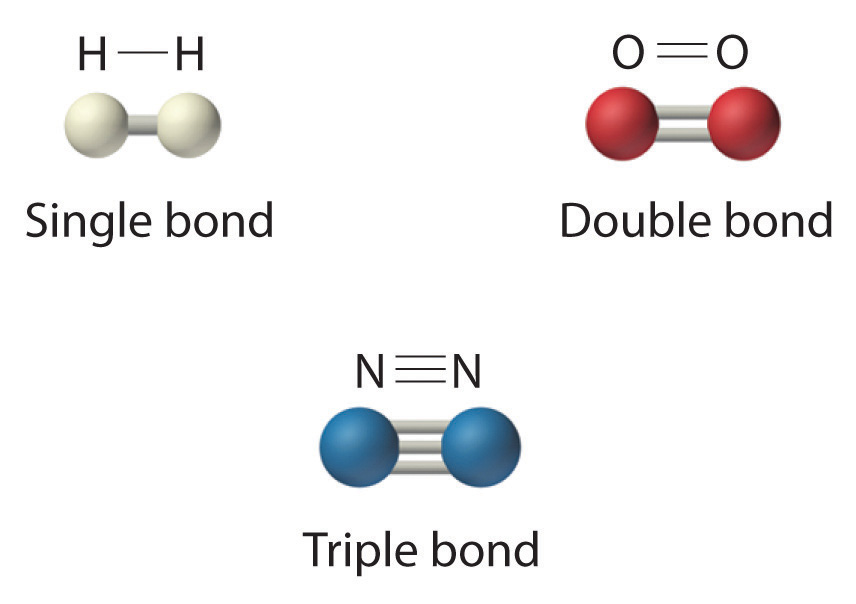Hydrogen (H2) has a single bond between atoms. Oxygen (O2) has a double bond between atoms, indicated by two lines (=). Nitrogen (N2) has a triple bond between atoms, indicated by three lines (≡). Each bond represents an electron pair.

Table 2.1 The Number of Bonds That Selected Atoms Commonly Form to Other Atoms

Atom Number of Bonds
H (group 1) 1
O (group 16) 2
N (group 15) 3
C (group 14) 4

The structural formula for water can be drawn as follows:Because the latter approximates the experimentally determined shape of the water molecule, it is more informative. Similarly, ammonia (NH3) and methane (CH4) are often written as planar molecules:As shown in Figure 2.3 "The Three-Dimensional Structures of Water, Ammonia, and Methane", however, the actual three-dimensional structure of NH3 looks like a pyramid with a triangular base of three hydrogen atoms. The structure of CH4, with four hydrogen atoms arranged around a central carbon atom as shown in Figure 2.3 "The Three-Dimensional Structures of Water, Ammonia, and Methane", is tetrahedral. That is, the hydrogen atoms are positioned at every other vertex of a cube. Many compounds—carbon compounds, in particular—have four bonded atoms arranged around a central atom to form a tetrahedron.

Figure 2.3 The Three-Dimensional Structures of Water, Ammonia, and Methane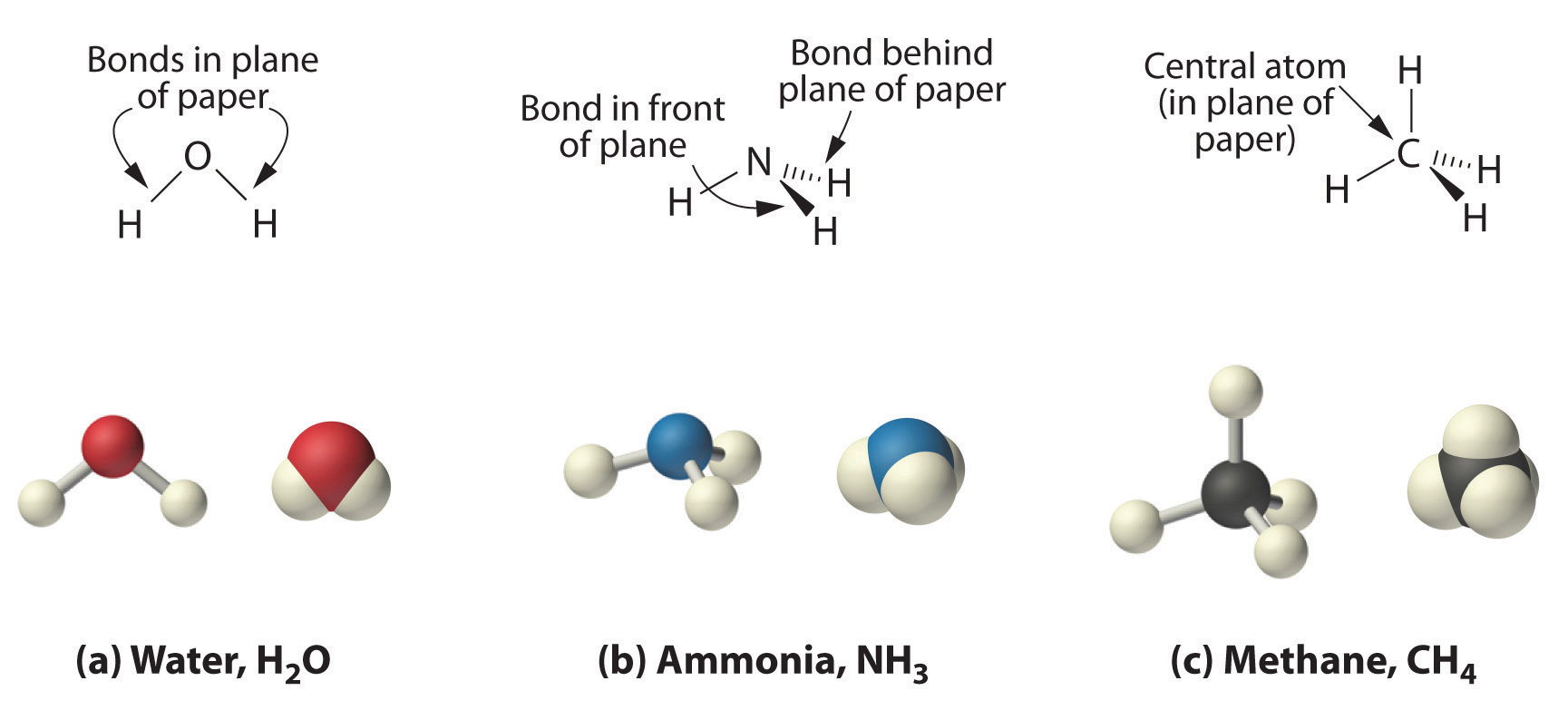(a) Water is a V-shaped molecule, in which all three atoms lie in a plane. (b) In contrast, ammonia has a pyramidal structure, in which the three hydrogen atoms form the base of the pyramid and the nitrogen atom is at the vertex. (c) The four hydrogen atoms of methane form a tetrahedron; the carbon atom lies in the center.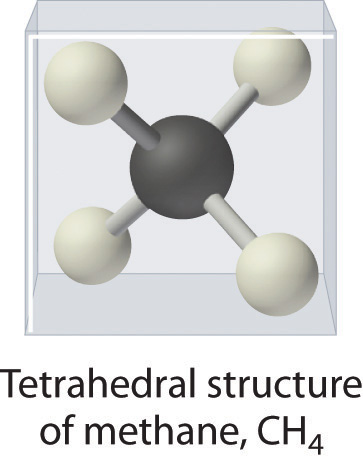CH4. Methane has a three-dimensional, tetrahedral structure.

Figure 2.1 "Elements That Exist as Covalent Molecules", Figure 2.2 "Molecules That Contain Single, Double, and Triple Bonds", and Figure 2.3 "The Three-Dimensional Structures of Water, Ammonia, and Methane" illustrate different ways to represent the structures of molecules. It should be clear that there is no single “best” way to draw the structure of a molecule; the method you use depends on which aspect of the structure you want to emphasize and how much time and effort you want to spend. Figure 2.4 "Different Ways of Representing the Structure of a Molecule" shows some of the different ways to portray the structure of a slightly more complex molecule: methanol. These representations differ greatly in their information content. For example, the molecular formula for methanol (part (a) in Figure 2.4 "Different Ways of Representing the Structure of a Molecule") gives only the number of each kind of atom; writing methanol as CH4O tells nothing about its structure. In contrast, the structural formula (part (b) in Figure 2.4 "Different Ways of Representing the Structure of a Molecule") indicates how the atoms are connected, but it makes methanol look as if it is planar (which it is not). Both the ball-and-stick model (part (c) in Figure 2.4 "Different Ways of Representing the Structure of a Molecule") and the perspective drawing (part (d) in Figure 2.4 "Different Ways of Representing the Structure of a Molecule") show the three-dimensional structure of the molecule. The latter (also called a wedge-and-dash representation) is the easiest way to sketch the structure of a molecule in three dimensions. It shows which atoms are above and below the plane of the paper by using wedges and dashes, respectively; the central atom is always assumed to be in the plane of the paper. The space-filling model (part (e) in Figure 2.4 "Different Ways of Representing the Structure of a Molecule") illustrates the approximate relative sizes of the atoms in the molecule, but it does not show the bonds between the atoms. Also, in a space-filling model, atoms at the “front” of the molecule may obscure atoms at the “back.”

Figure 2.4 Different Ways of Representing the Structure of a Molecule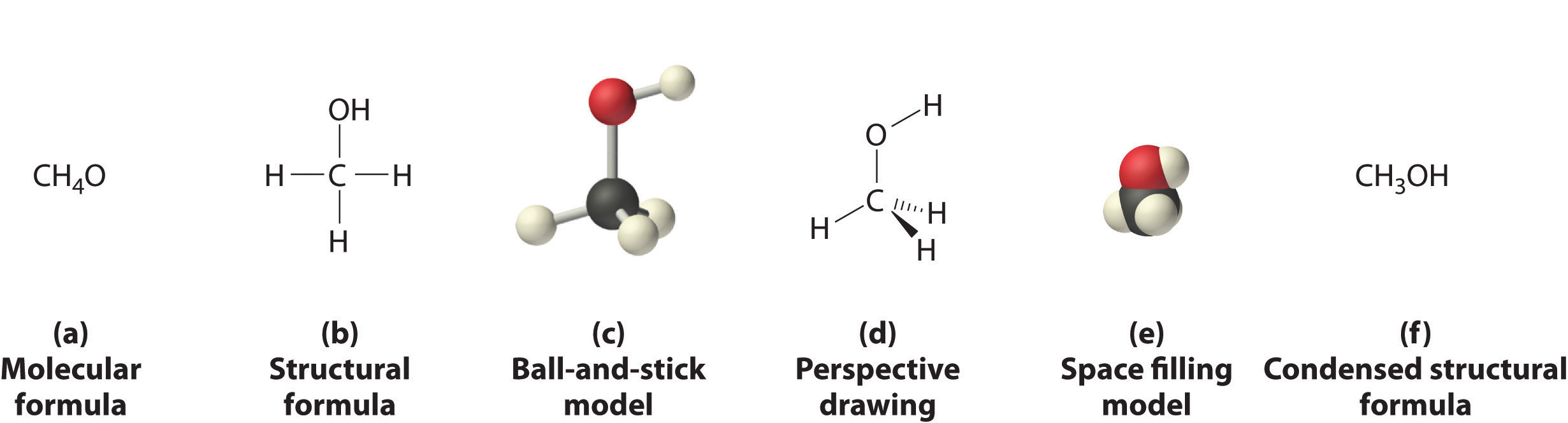(a) The molecular formula for methanol gives only the number of each kind of atom present. (b) The structural formula shows which atoms are connected. (c) The ball-and-stick model shows the atoms as spheres and the bonds as sticks. (d) A perspective drawing (also called a wedge-and-dash representation) attempts to show the three-dimensional structure of the molecule. (e) The space-filling model shows the atoms in the molecule but not the bonds. (f) The condensed structural formula is by far the easiest and most common way to represent a molecule.

Although a structural formula, a ball-and-stick model, a perspective drawing, and a space-filling model provide a significant amount of information about the structure of a molecule, each requires time and effort. Consequently, chemists often use a condensed structural formula (part (f) in Figure 2.4 "Different Ways of Representing the Structure of a Molecule"), which omits the lines representing bonds between atoms and simply lists the atoms bonded to a given atom next to it. Multiple groups attached to the same atom are shown in parentheses, followed by a subscript that indicates the number of such groups. For example, the condensed structural formula for methanol is CH3OH, which tells us that the molecule contains a CH3 unit that looks like a fragment of methane (CH4). Methanol can therefore be viewed either as a methane molecule in which one hydrogen atom has been replaced by an –OH group or as a water molecule in which one hydrogen atom has been replaced by a –CH3 fragment. Because of their ease of use and information content, we use condensed structural formulas for molecules throughout this text. Ball-and-stick models are used when needed to illustrate the three-dimensional structure of molecules, and space-filling models are used only when it is necessary to visualize the relative sizes of atoms or molecules to understand an important point.

### Example 2

Write the molecular formula for each compound. The condensed structural formula is given.

1. Sulfur monochloride (also called disulfur dichloride) is a vile-smelling, corrosive yellow liquid used in the production of synthetic rubber. Its condensed structural formula is ClSSCl.
2. Ethylene glycol is the major ingredient in antifreeze. Its condensed structural formula is HOCH2CH2OH.
3. Trimethylamine is one of the substances responsible for the smell of spoiled fish. Its condensed structural formula is (CH3)3N.

Given: condensed structural formula

Asked for: molecular formula

Strategy:

A Identify every element in the condensed structural formula and then determine whether the compound is organic or inorganic.

B As appropriate, use either organic or inorganic convention to list the elements. Then add appropriate subscripts to indicate the number of atoms of each element present in the molecular formula.

Solution:

The molecular formula lists the elements in the molecule and the number of atoms of each.

1. A Each molecule of sulfur monochloride has two sulfur atoms and two chlorine atoms. Because it does not contain mostly carbon and hydrogen, it is an inorganic compound. B Sulfur lies to the left of chlorine in the periodic table, so it is written first in the formula. Adding subscripts gives the molecular formula S2Cl2.
2. A Counting the atoms in ethylene glycol, we get six hydrogen atoms, two carbon atoms, and two oxygen atoms per molecule. The compound consists mostly of carbon and hydrogen atoms, so it is organic. B As with all organic compounds, C and H are written first in the molecular formula. Adding appropriate subscripts gives the molecular formula C2H6O2.
3. A The condensed structural formula shows that trimethylamine contains three CH3 units, so we have one nitrogen atom, three carbon atoms, and nine hydrogen atoms per molecule. Because trimethylamine contains mostly carbon and hydrogen, it is an organic compound. B According to the convention for organic compounds, C and H are written first, giving the molecular formula C3H9N.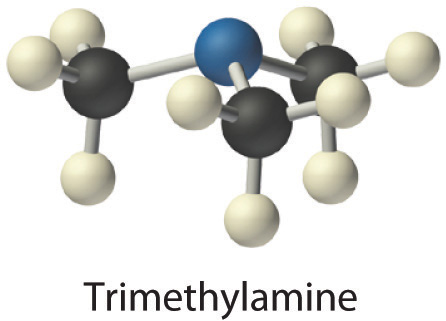Exercise

Write the molecular formula for each molecule.

1. Chloroform, which was one of the first anesthetics and was used in many cough syrups until recently, contains one carbon atom, one hydrogen atom, and three chlorine atoms. Its condensed structural formula is CHCl3.
2. Hydrazine is used as a propellant in the attitude jets of the space shuttle. Its condensed structural formula is H2NNH2.
3. Putrescine is a pungent-smelling compound first isolated from extracts of rotting meat. Its condensed structural formula is H2NCH2CH2CH2CH2NH2. This is often written as H2N(CH2)4NH2 to indicate that there are four CH2 fragments linked together.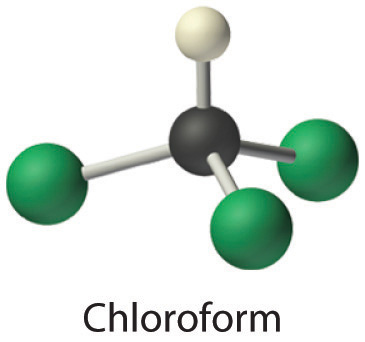Answer:

1. CHCl3
2. N2H4
3. C4H12N2

## Ionic Compounds

The substances described in the preceding discussion are composed of molecules that are electrically neutral; that is, the number of positively charged protons in the nucleus is equal to the number of negatively charged electrons. In contrast, ions are atoms or assemblies of atoms that have a net electrical charge. Ions that contain fewer electrons than protons have a net positive charge and are called cationsAn ion that has fewer electrons than protons, resulting in a net positive charge.. Conversely, ions that contain more electrons than protons have a net negative charge and are called anionsAn ion that has fewer protons than electrons, resulting in a net negative charge.. Ionic compounds contain both cations and anions in a ratio that results in no net electrical charge.

### Note the Pattern

Ionic compounds contain both cations and anions in a ratio that results in zero electrical charge.

In covalent compounds, electrons are shared between bonded atoms and are simultaneously attracted to more than one nucleus. In contrast, ionic compounds contain cations and anions rather than discrete neutral molecules. Ionic compounds are held together by the attractive electrostatic interactions between cations and anions. In an ionic compound, the cations and anions are arranged in space to form an extended three-dimensional array that maximizes the number of attractive electrostatic interactions and minimizes the number of repulsive electrostatic interactions (Figure 2.5 "Covalent and Ionic Bonding"). As shown in Equation 2.1, the electrostatic energy of the interaction between two charged particles is proportional to the product of the charges on the particles and inversely proportional to the distance between them:

Equation 2.1

where Q1 and Q2 are the electrical charges on particles 1 and 2, and r is the distance between them. When Q1 and Q2 are both positive, corresponding to the charges on cations, the cations repel each other and the electrostatic energy is positive. When Q1 and Q2 are both negative, corresponding to the charges on anions, the anions repel each other and the electrostatic energy is again positive. The electrostatic energy is negative only when the charges have opposite signs; that is, positively charged species are attracted to negatively charged species and vice versa. As shown in Figure 2.6 "The Effect of Charge and Distance on the Strength of Electrostatic Interactions", the strength of the interaction is proportional to the magnitude of the charges and decreases as the distance between the particles increases. We will return to these energetic factors in Chapter 8 "Ionic versus Covalent Bonding", where they are described in greater quantitative detail.

### Note the Pattern

If the electrostatic energy is positive, the particles repel each other; if the electrostatic energy is negative, the particles are attracted to each other.

Figure 2.5 Covalent and Ionic Bonding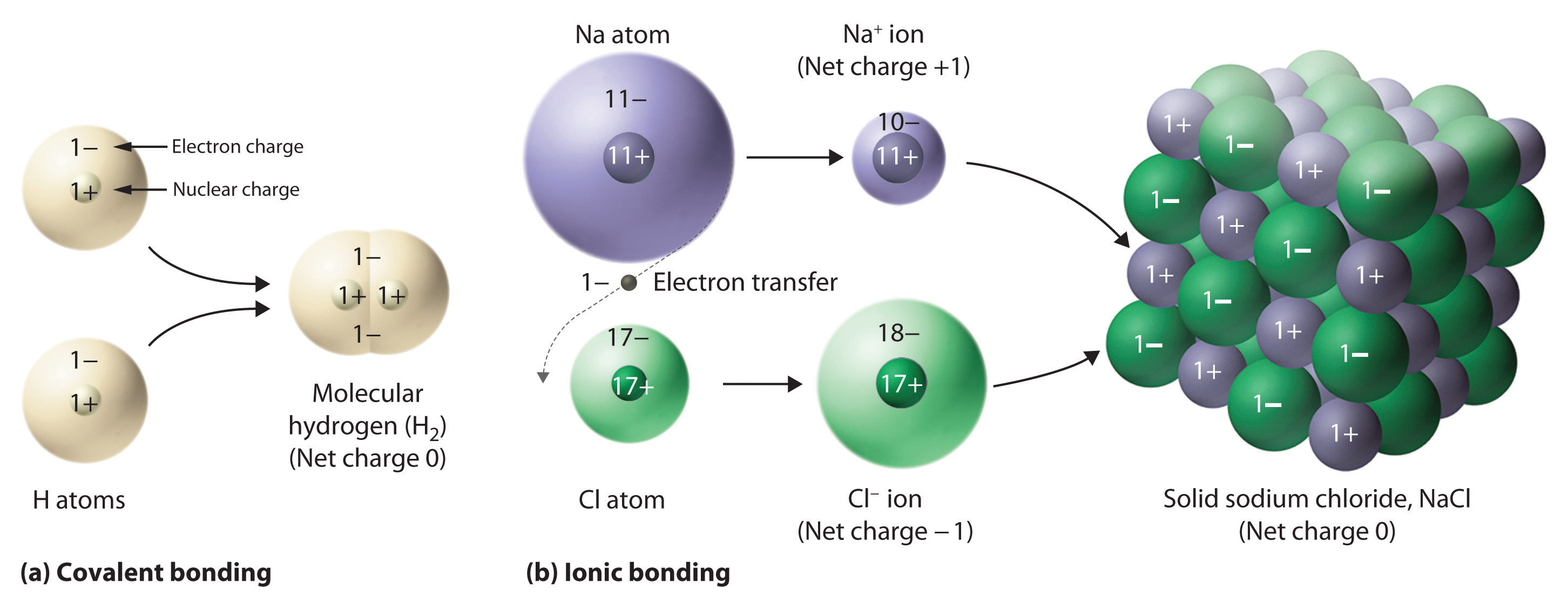(a) In molecular hydrogen (H2), two hydrogen atoms share two electrons to form a covalent bond. (b) The ionic compound NaCl forms when electrons from sodium atoms are transferred to chlorine atoms. The resulting Na+ and Cl ions form a three-dimensional solid that is held together by attractive electrostatic interactions.

Figure 2.6 The Effect of Charge and Distance on the Strength of Electrostatic Interactions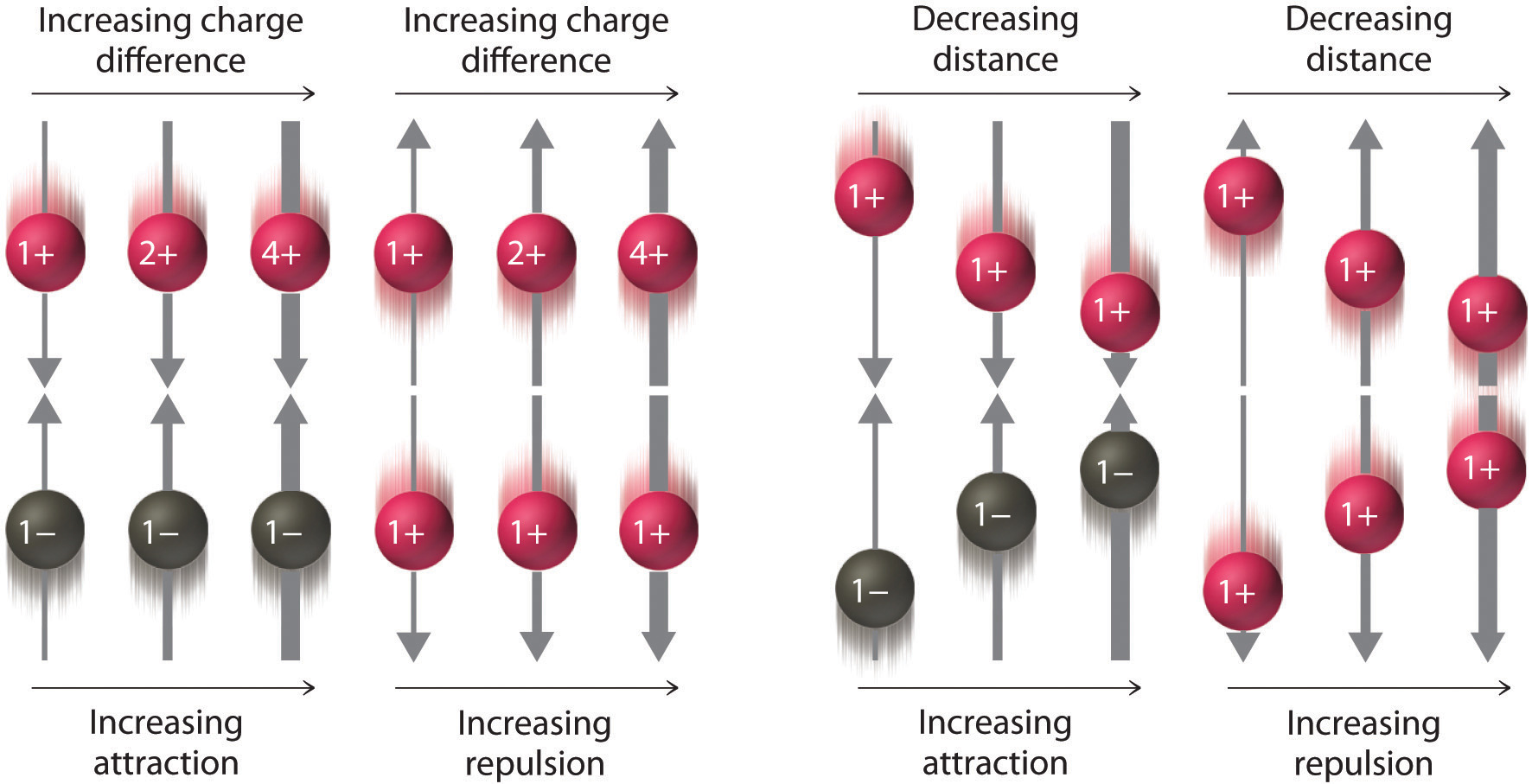As the charge on ions increases or the distance between ions decreases, so does the strength of the attractive (−…+) or repulsive (−…− or +…+) interactions. The strength of these interactions is represented by the thickness of the arrows.

One example of an ionic compound is sodium chloride (NaCl; Figure 2.7 "Sodium Chloride: an Ionic Solid"), formed from sodium and chlorine. In forming chemical compounds, many elements have a tendency to gain or lose enough electrons to attain the same number of electrons as the noble gas closest to them in the periodic table. When sodium and chlorine come into contact, each sodium atom gives up an electron to become a Na+ ion, with 11 protons in its nucleus but only 10 electrons (like neon), and each chlorine atom gains an electron to become a Cl ion, with 17 protons in its nucleus and 18 electrons (like argon), as shown in part (b) in Figure 2.5 "Covalent and Ionic Bonding". Solid sodium chloride contains equal numbers of cations (Na+) and anions (Cl), thus maintaining electrical neutrality. Each Na+ ion is surrounded by 6 Cl ions, and each Cl ion is surrounded by 6 Na+ ions. Because of the large number of attractive Na+Cl interactions, the total attractive electrostatic energy in NaCl is great.

Figure 2.7 Sodium Chloride: an Ionic Solid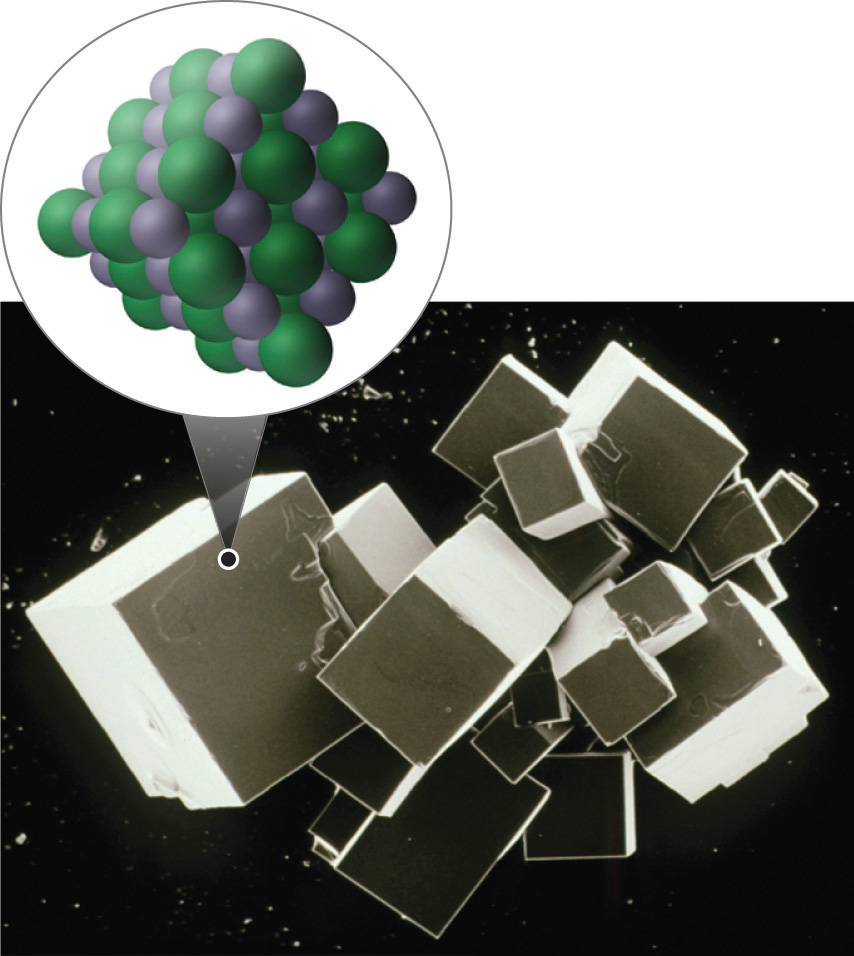The planes of an NaCl crystal reflect the regular three-dimensional arrangement of its Na+ (purple) and Cl (green) ions.

Consistent with a tendency to have the same number of electrons as the nearest noble gas, when forming ions, elements in groups 1, 2, and 3 tend to lose one, two, and three electrons, respectively, to form cations, such as Na+ and Mg2+. They then have the same number of electrons as the nearest noble gas: neon. Similarly, K+, Ca2+, and Sc3+ have 18 electrons each, like the nearest noble gas: argon. In addition, the elements in group 13 lose three electrons to form cations, such as Al3+, again attaining the same number of electrons as the noble gas closest to them in the periodic table. Because the lanthanides and actinides formally belong to group 3, the most common ion formed by these elements is M3+, where M represents the metal. Conversely, elements in groups 17, 16, and 15 often react to gain one, two, and three electrons, respectively, to form ions such as Cl, S2−, and P3−. Ions such as these, which contain only a single atom, are called monatomic ionsAn ion with only a single atom.. You can predict the charges of most monatomic ions derived from the main group elements by simply looking at the periodic table and counting how many columns an element lies from the extreme left or right. For example, you can predict that barium (in group 2) will form Ba2+ to have the same number of electrons as its nearest noble gas, xenon, that oxygen (in group 16) will form O2− to have the same number of electrons as neon, and cesium (in group 1) will form Cs+ to also have the same number of electrons as xenon. Note that this method does not usually work for most of the transition metals, as you will learn in Section 2.3 "Naming Ionic Compounds". Some common monatomic ions are in Table 2.2 "Some Common Monatomic Ions and Their Names".

### Note the Pattern

Elements in groups 1, 2, and 3 tend to form 1+, 2+, and 3+ ions, respectively; elements in groups 15, 16, and 17 tend to form 3−, 2−, and 1− ions, respectively.

Table 2.2 Some Common Monatomic Ions and Their Names

Group 1 Group 2 Group 3 Group 13 Group 15 Group 16 Group 17

Li+

lithium

Be2+

beryllium

N3−

nitride

(azide)

O2−

oxide

F

fluoride

Na+

sodium

Mg2+

magnesium

Al3+

aluminum

P3−

phosphide

S2−

sulfide

Cl

chloride

K+

potassium

Ca2+

calcium

Sc3+

scandium

Ga3+

gallium

As3−

arsenide

Se2−

selenide

Br

bromide

Rb+

rubidium

Sr2+

strontium

Y3+

yttrium

In3+

indium

Te2−

telluride

I

iodide

Cs+

cesium

Ba2+

barium

La3+

lanthanum

### Example 3

Predict the charge on the most common monatomic ion formed by each element.

1. aluminum, used in the quantum logic clock, the world’s most precise clock
2. selenium, used to make ruby-colored glass
3. yttrium, used to make high-performance spark plugs

Given: element

Asked for: ionic charge

Strategy:

A Identify the group in the periodic table to which the element belongs. Based on its location in the periodic table, decide whether the element is a metal, which tends to lose electrons; a nonmetal, which tends to gain electrons; or a semimetal, which can do either.

B After locating the noble gas that is closest to the element, determine the number of electrons the element must gain or lose to have the same number of electrons as the nearest noble gas.

Solution:

1. A Aluminum is a metal in group 13; consequently, it will tend to lose electrons. B The nearest noble gas to aluminum is neon. Aluminum will lose three electrons to form the Al3+ ion, which has the same number of electrons as neon.
2. A Selenium is a nonmetal in group 16, so it will tend to gain electrons. B The nearest noble gas is krypton, so we predict that selenium will gain two electrons to form the Se2− ion, which has the same number of electrons as krypton.
3. A Yttrium is in group 3, and elements in this group are metals that tend to lose electrons. B The nearest noble gas to yttrium is krypton, so yttrium is predicted to lose three electrons to form Y3+, which has the same number of electrons as krypton.

Exercise

Predict the charge on the most common monatomic ion formed by each element.

1. calcium, used to prevent osteoporosis
2. iodine, required for the synthesis of thyroid hormones
3. zirconium, widely used in nuclear reactors

Answer:

1. Ca2+
2. I
3. Zr4+

## Physical Properties of Ionic and Covalent Compounds

In general, ionic and covalent compounds have different physical properties. Ionic compounds usually form hard crystalline solids that melt at rather high temperatures and are very resistant to evaporation. These properties stem from the characteristic internal structure of an ionic solid, illustrated schematically in part (a) in Figure 2.8 "Interactions in Ionic and Covalent Solids", which shows the three-dimensional array of alternating positive and negative ions held together by strong electrostatic attractions. In contrast, as shown in part (b) in Figure 2.8 "Interactions in Ionic and Covalent Solids", most covalent compounds consist of discrete molecules held together by comparatively weak intermolecular forces (the forces between molecules), even though the atoms within each molecule are held together by strong intramolecular covalent bonds (the forces within the molecule). Covalent substances can be gases, liquids, or solids at room temperature and pressure, depending on the strength of the intermolecular interactions. Covalent molecular solids tend to form soft crystals that melt at rather low temperatures and evaporate relatively easily.Some covalent substances, however, are not molecular but consist of infinite three-dimensional arrays of covalently bonded atoms and include some of the hardest materials known, such as diamond. This topic will be addressed in Chapter 12 "Solids". The covalent bonds that hold the atoms together in the molecules are unaffected when covalent substances melt or evaporate, so a liquid or vapor of discrete, independent molecules is formed. For example, at room temperature, methane, the major constituent of natural gas, is a gas that is composed of discrete CH4 molecules. A comparison of the different physical properties of ionic compounds and covalent molecular substances is given in Table 2.3 "The Physical Properties of Typical Ionic Compounds and Covalent Molecular Substances".

Table 2.3 The Physical Properties of Typical Ionic Compounds and Covalent Molecular Substances

Ionic Compounds Covalent Molecular Substances
hard solids gases, liquids, or soft solids
high melting points low melting points
nonvolatile volatile

Figure 2.8 Interactions in Ionic and Covalent Solids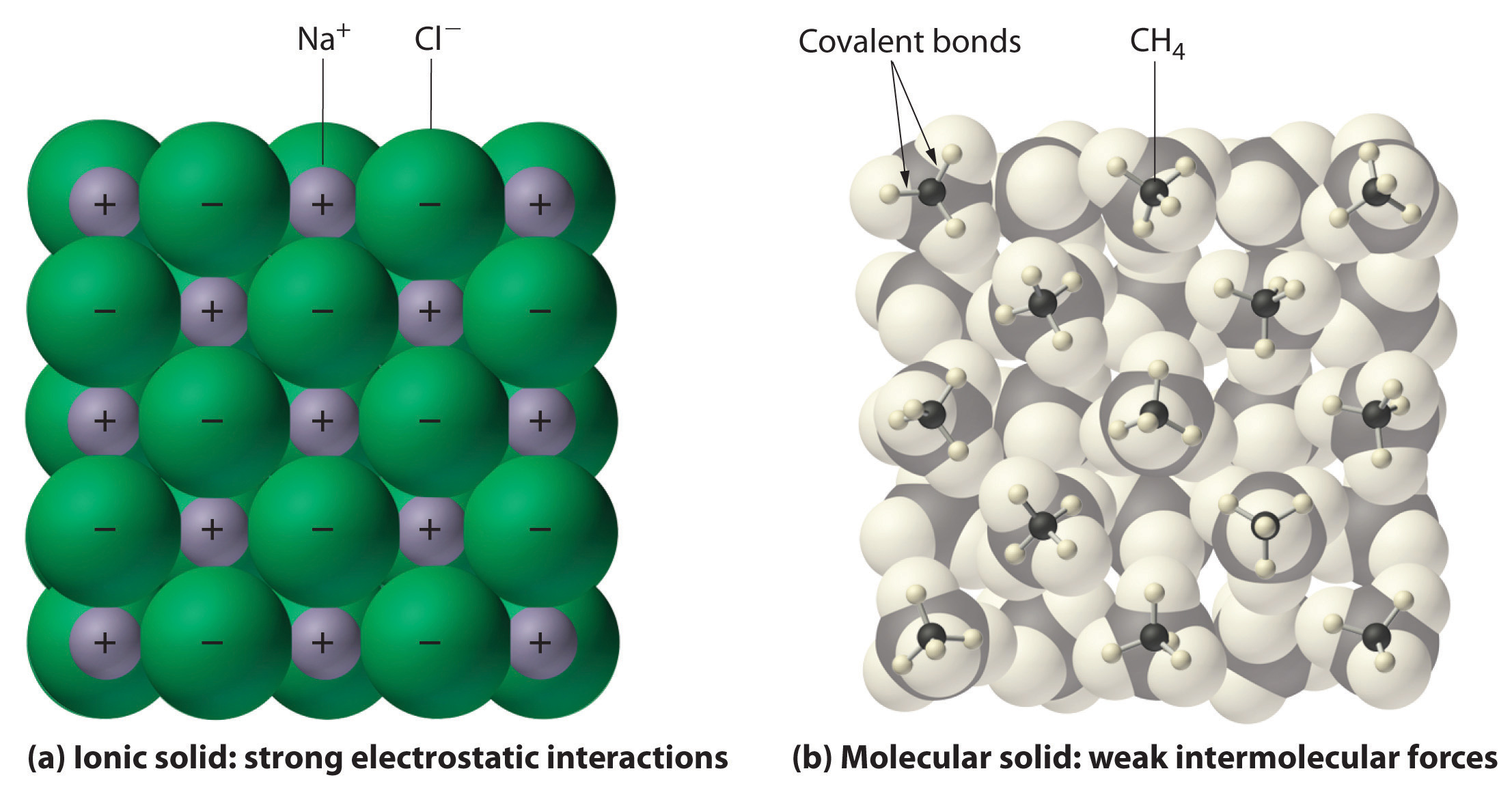(a) The positively and negatively charged ions in an ionic solid such as sodium chloride (NaCl) are held together by strong electrostatic interactions. (b) In this representation of the packing of methane (CH4) molecules in solid methane, a prototypical molecular solid, the methane molecules are held together in the solid only by relatively weak intermolecular forces, even though the atoms within each methane molecule are held together by strong covalent bonds.

### Summary

The atoms in chemical compounds are held together by attractive electrostatic interactions known as chemical bonds. Ionic compounds contain positively and negatively charged ions in a ratio that results in an overall charge of zero. The ions are held together in a regular spatial arrangement by electrostatic forces. Most covalent compounds consist of molecules, groups of atoms in which one or more pairs of electrons are shared by at least two atoms to form a covalent bond. The atoms in molecules are held together by the electrostatic attraction between the positively charged nuclei of the bonded atoms and the negatively charged electrons shared by the nuclei. The molecular formula of a covalent compound gives the types and numbers of atoms present. Compounds that contain predominantly carbon and hydrogen are called organic compounds, whereas compounds that consist primarily of elements other than carbon and hydrogen are inorganic compounds. Diatomic molecules contain two atoms, and polyatomic molecules contain more than two. A structural formula indicates the composition and approximate structure and shape of a molecule. Single bonds, double bonds, and triple bonds are covalent bonds in which one, two, and three pairs of electrons, respectively, are shared between two bonded atoms. Atoms or groups of atoms that possess a net electrical charge are called ions; they can have either a positive charge (cations) or a negative charge (anions). Ions can consist of one atom (monatomic ions) or several (polyatomic ions). The charges on monatomic ions of most main group elements can be predicted from the location of the element in the periodic table. Ionic compounds usually form hard crystalline solids with high melting points. Covalent molecular compounds, in contrast, consist of discrete molecules held together by weak intermolecular forces and can be gases, liquids, or solids at room temperature and pressure.

### Key Takeaway

• There are two fundamentally different kinds of chemical bonds (covalent and ionic) that cause substances to have very different properties.

### Conceptual Problems

1. Ionic and covalent compounds are held together by electrostatic attractions between oppositely charged particles. Describe the differences in the nature of the attractions in ionic and covalent compounds. Which class of compounds contains pairs of electrons shared between bonded atoms?

2. Which contains fewer electrons than the neutral atom—the corresponding cation or the anion?

3. What is the difference between an organic compound and an inorganic compound?

4. What is the advantage of writing a structural formula as a condensed formula?

5. The majority of elements that exist as diatomic molecules are found in one group of the periodic table. Identify the group.

6. Discuss the differences between covalent and ionic compounds with regard to

1. the forces that hold the atoms together.
2. melting points.
3. physical states at room temperature and pressure.
7. Why do covalent compounds generally tend to have lower melting points than ionic compounds?

### Answer

1. Covalent compounds generally melt at lower temperatures than ionic compounds because the intermolecular interactions that hold the molecules together in a molecular solid are weaker than the electrostatic attractions that hold oppositely charged ions together in an ionic solid.

### Numerical Problems

1. The structural formula for chloroform (CHCl3) was shown in Example 2. Based on this information, draw the structural formula of dichloromethane (CH2Cl2).

2. What is the total number of electrons present in each ion?

1. F
2. Rb+
3. Ce3+
4. Zr4+
5. Zn2+
6. Kr2+
7. B3+
3. What is the total number of electrons present in each ion?

1. Ca2+
2. Se2−
3. In3+
4. Sr2+
5. As3+
6. N3−
7. Tl+
4. Predict how many electrons are in each ion.

1. an oxygen ion with a −2 charge
2. a beryllium ion with a +2 charge
3. a silver ion with a +1 charge
4. a selenium ion with a +4 charge
5. an iron ion with a +2 charge
6. a chlorine ion with a −1 charge
5. Predict how many electrons are in each ion.

1. a copper ion with a +2 charge
2. a molybdenum ion with a +4 charge
3. an iodine ion with a −1 charge
4. a gallium ion with a +3 charge
5. an ytterbium ion with a +3 charge
6. a scandium ion with a +3 charge
6. Predict the charge on the most common monatomic ion formed by each element.

1. chlorine
2. phosphorus
3. scandium
4. magnesium
5. arsenic
6. oxygen
7. Predict the charge on the most common monatomic ion formed by each element.

1. sodium
2. selenium
3. barium
4. rubidium
5. nitrogen
6. aluminum
8. For each representation of a monatomic ion, identify the parent atom, write the formula of the ion using an appropriate superscript, and indicate the period and group of the periodic table in which the element is found.

1. $X 4 9 2+$
2. $X 1 1 –$
9. For each representation of a monatomic ion, identify the parent atom, write the formula of the ion using an appropriate superscript, and indicate the period and group of the periodic table in which the element is found.

1. $X 3 7 +$
2. $X 13 27 3+$

### Answers

1. 27
2. 38
3. 54
4. 28
5. 67
6. 18
1. Li, Li+, 2nd period, group 1
2. F, F, 2nd period, group 17
3. Al, Al3+, 3nd period, group 13